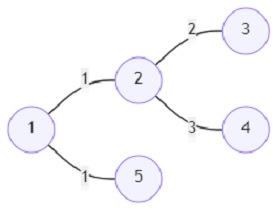# Program to find out the minimum path to deliver all letters in Python

Suppose there are n cities and that are connected with n -1 roads. A city can be visited from any other city. Now the postal system of the cities delivers k letters daily. The letter's destination can be any of the k different cities. A postal worker has to deliver all the letters to their addresses each day. We shall have to find out the minimum distance the worker has to travel to deliver all the letters. The worker can start from any given city.

So, if the input is likeand the letters have to be delivered in cities (delv) 1, 2, and 4; then the output will be 4.

The worker can start delivering either from cities 1, 2, or 4. If the worker starts at city 1, then the path will be 1->2->4, vice-versa in the case of city 4; 4->2->1. The total cost will be 1 + 3 = 4. If he starts from city 2, the cost will be greater than the other two.

To solve this, we will follow these steps −

• Define a function depth_search() . This will take node, p
• d1 := -infinity
• d2 := -infinity
• for each pair x, y in adj_list[node], do
• if x is not same as p, then
• d1 := maximum of (d1, depth_search(x, node) + y)
• if d1 > d2, then
• swap the values of d2 and d1
• ti[node] := ti[node] + ti[x]
• if 0 < ti[x] < k, then
• SUM := SUM + y
• if d1 > 0, then
• MAX := maximum of (MAX, d1 + d2)
• if d2 > 0 and tj[node] is non-zero, then
• MAX := maximum of(MAX, d2)
• if tj[node] is non-zero, then
• d2 := max(0, d2)
• return d2
• k := size of delv
• adj_list := a new map
• ti := a new list of size (nodes + 5) initialized with 0
• tj := a new list of size (nodes + 5) initialized with 0
• for each i in delv, do
• ti[i] := 1
• tj[i] := 1
• for each item in roads, do
• x := item
• y := item
• c := item
• if x is not present in adj_list, then
• if y is not present in adj_list, then
• append (y, c) at the end of adj_list[x]
• append (x, c) at the end of adj_list[y]
• SUM := 0
• MAX := 0
• depth_search(1, 1)
• return SUM * 2 - MAX

## Example

Let us see the following implementation to get better understanding −

import sys
from math import inf as INF
sys.setrecursionlimit(10**5 + 5)

def depth_search(node, p):
global SUM, MAX
d1 = -INF
d2 = -INF

if x != p:
d1 = max(d1, depth_search(x, node) + y)
if d1 > d2:
d1, d2 = d2, d1

ti[node] += ti[x]
if 0 < ti[x] < k:
SUM += y

if d1 > 0: MAX = max(MAX, d1 + d2)
if d2 > 0 and tj[node]: MAX = max(MAX, d2)
if tj[node]: d2 = max(0, d2)

return d2

global k, ti, tj, adj_list, SUM, MAX
k = len(delv)
ti =  * (nodes + 5)
tj =  * (nodes + 5)

for i in delv:
ti[i] = tj[i] = 1

x, y, c = map(int, item)

SUM = 0
MAX = 0
depth_search(1,1)
return SUM * 2 - MAX

print(solve(5, [1, 2, 4], [(1,2,1),(2,3,2),(2,4,3),(1,5,1)]))


## Input

5, [1, 2, 4], [(1,2,1),(2,3,2),(2,4,3),(1,5,1)]

## Output

4